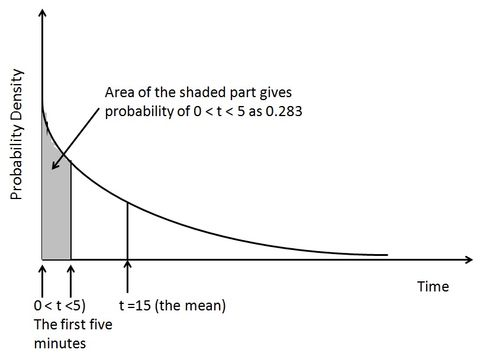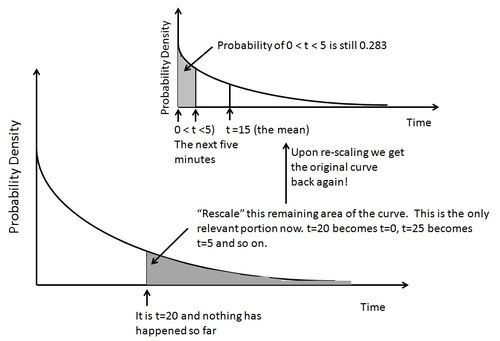# Unconditioned by the past

by Hari Balasubramanian
Exploring the Memoryless property of the Exponential Distribution.
Waiting For the Next Customer
Suppose you run a small business, a barber shop or a small restaurant that takes walk-ins only. A customer has just left, your place is empty, and you are waiting for the next customer to come in. You've figured out that on average the time between two successive arrivals is 15 minutes. However, there is variation and the variation follows the Exponential probability curve shown in the figure below. This is not an arbitrary choice: time between successive random and independent arrivals does actually follow the Exponential. The average time between arrivals depends on whether it is a busy or slow time of the day, but the general shape of the Exponential curve keeps showing up again and again when empirical data is plotted (one example here).The height of the curve is an indicator of where the greatest probability densities are. Most arrivals happen in quick succession (the curve is tall when t is small), but there will be occasions when a long time elapses before the next arrival happens. At t=0, when the last customer just left, if you calculated the probability of the next customer arriving within 5 minutes (0 < t < 5) you would get the value 0.283. Equivalently you could say that the probability you will wait 5 minutes or more is (1 - 0.283) = 0.717.

Now here's the interesting part. Suppose twenty minutes have now passed and the next customer still hasn't arrived. You are starting to get a little impatient; after all you don't want your productive time to be idle. So at t=20, you again calculate the probability of a customer arriving in the next 5 minutes (20 < t < 25), given that no one has come so far. You would think this new probability, based on how much time has elapsed, should be higher than 0.283. But, surprisingly, the probability that a customer will arrive in the next 5 minutes, given that twenty idle minutes have passed, is still 0.283! And the probability that you will wait 5 minutes or more is still 0.717.

This is precisely the Memoryless property of the Exponential: the past has been forgotten; the probability of when the next event will happen remains unconditioned by when the last event happened. Fast forward even more: let's say you've waited for half an hour. No one has shown up so far. Frustrated, you recalculate the probability of someone arriving in the next 5 minutes (30 < t < 35). Still 0.283!

The behavior that we see in the Exponential is because your customers are arriving independently of one another — remember that you allow only walk-ins. There is no “memory” or predetermined schedule connecting any two successive arrivals (in the same way that the outcome of a coin that is tossed now has no memory or connection with the outcome of a coin tossed at some point of time in the past). A barber shop, a small restaurant, a shoe-shop, a cab-driver, a car mechanic, a self-employed person who earns a living doing Japanese-English translation requests – one can find many contexts that experience the Memoryless property. Bigger retail firms also experience the same problem, but they hire (and fire) many people, cross-train their employees to do multiple tasks and thereby have ways to reduce the risk of staying idle.

In small businesses, the wait for the next customer is felt far more personally and acutely. Recently, I spoke to Abel (not his real name), an Ethiopian man who had started a restaurant in a small Midwestern town. The Ethiopian dishes I tasted were excellent. Yet Abel said there were many difficult evenings he would be alone, waiting for someone to come in. To cut costs, he was both the cook and the server on such slow days. But Abel noted that he would, unexpectedly, get busy. This is the flip side of the Exponential: a string of closely spaced arrivals is very likely since the probability densities are front heavy, as seen in the shape of the curve. So you can go from being idle for an hour to suddenly having a long line of people waiting. Now you have a different problem – you are too busy and your customers are unhappy!

A Visual Illustration

Let's look closer why exactly the Memoryless property holds true for the Exponential. Instead of showing the algebra, I'll try illustrating visually. I struggled with the Memoryless property myself for many years; so at the very least, I'll put my own thoughts in order. Please let me know if something does not sound right.

The Exponential is a continuous distribution used to characterize the probability of time durations, such as the time between two successive randomly occurring events. Naturally the smallest possible value is 0. The Exponential curve is asymptotic – a fancy word for the idea that the probability curve keeps dipping as we move to the right and gets closer and closer to the x axis, but never quite dips enough to touch the x-axis. The dipping curve stretches to infinity. So very long time between events (long periods of idleness) are theoretically possible, although in practice they are very, very unlikely. The area under the Exponential probability curve, if we calculate the limit, tends to 1 (as it must for any continuous probability distribution).Let's return to the original example. Time between successive arrivals follows an Exponential Distribution with a mean of 15 minutes. Currently, twenty minutes have passed since the last arrival, so we are at t=20. We are trying to find out the probability that an arrival will happen in the next 5 minutes — in the interval 20 < t < 25. To do so, we now only need to consider the area under the Exponential curve to the right of the t=20 mark. The total area under the curve to the right of t=20 is 0.263. We “rescale” this area such that 0.263 now becomes equivalent to an area of 1 — we do this because this is the relevant conditional probability space we are now interested in. Further, the x axis is re-scaled: t=20 becomes t=0; t=25 becomes t=5; and so on, so that in the newly re-scaled or conditioned area we can calculate the probability of an event happening in the next 5 minutes.

Surprisingly, the re-scaled area is exactly the original Exponential probability curve! Even the height of the curve corresponding to every time value on the x-axis is exactly as it was when the last customer left.

It's not a precise analogy, but just as the same pattern keeps repeating itself in a fractal no matter how much you magnify the original, so the same exact Exponential curve we started with keeps appearing again and again upon re-scaling no matter how much time has elapsed. It does not matter if 10, 30, 100 or 2000 minutes have passed without an arrival; the probability that an arrival will happen in the next five minutes will always be 0.283. This makes mathematical calculations very straightforward — the past does not need to be kept track of, and the same formulas can be used at any stage.

There is something about how the curve decays or dips, more specifically the rate at which it decays, which gives Exponential this unique property among continuous probability distributions. In fact, if you knew that time durations follow the Memoryless property you can work backwards and prove that the original probability curve has to be Exponential.

As a contrast, other well known continuous distributions, say the Normal or Lognormal, do not have the Memoryless property. The Lognormal distribution is a more relevant comparison since, like the Exponential, it allows only values greater than 0, unlike the normal which allows negative values and is therefore not always appropriate for modeling time durations. In the Lognormal and Normal, the probability of a future event is not unconditioned by how much time has passed. This means that you have to keep track of the past when you calculate the possibility of a future event — and this quickly gets very cumbersome and computationally expensive.

For a further contrast, I've created a couple of roughly equivalent images, Figure 1 and Figure 2, for the continuous uniform distribution — a relatively simple, bounded distribution with a flat curve. Here we see that the probability of an event happening in the next five minutes was originally 0.1667; after twenty minutes, it went up to 0.5.

Among discrete distributions, the Geometric distribution has the Memoryless property.

Lifetime of a Device

I'd like to end the piece by raising a couple of questions. Probability textbooks routinely mention that the Exponential distribution can be used to model the lifetime of a device: time from when the device is put into operation to its failure. Here the Memoryless property seems puzzling to me.

If a device has worked for 3000 hours, the probability that it will work for another 1000 hours is exactly the same as when the device started operating. I find that quite amazing. Such a property is possible only if the failure of the device has nothing to do with wear and tear caused due to time. Otherwise, the longer the device works, the more likely it is to fail in the next time interval — just as at the age of 70, the probability that we will die in the next 10 years is much higher than the same probability calculated at the age of 40. From what I've read, the lifetime of semiconductor components follows exponential time to failure distributions. But then how is it that these devices escape wear and tear caused due to time? And are there other examples?